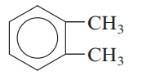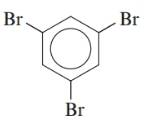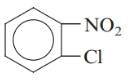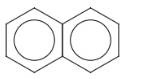Chapter 20, Problem 135AP### Introductory Chemistry: A Foundati...

9th Edition
Steven S. Zumdahl + 1 other
ISBN: 9781337399425

#### Solutions

Chapter
Section### Introductory Chemistry: A Foundati...

9th Edition
Steven S. Zumdahl + 1 other
ISBN: 9781337399425
Textbook Problem
1 views

# . Name each of the following aromatic or substituted aromatic compounds. a.b.c.d.Interpretation Introduction

(a)

Interpretation:

Name of the given substituted aromatic compound should be given.

Concept Introduction:

Name of benzene substituted aromatic compounds are done with suffix of benzene.

Explanation

The given compound is:

There are two methyl substituents in benzene ring...

Interpretation Introduction

(b)

Interpretation:

Name of the given substituted aromatic compound should be given.

Concept Introduction:

Name of benzene substituted aromatic compounds are done with suffix of benzene.

Interpretation Introduction

(c)

Interpretation:

Name of the given substituted aromatic compound should be given.

Concept Introduction:

Name of benzene substituted aromatic compounds are done with suffix of benzene.

Interpretation Introduction

(d)

Interpretation:

Name of the given aromatic compound should be given.

Concept Introduction:

The given compound is a fused system in which two benzene rings are fused.

### Still sussing out bartleby?

Check out a sample textbook solution.

See a sample solution

#### The Solution to Your Study Problems

Bartleby provides explanations to thousands of textbook problems written by our experts, many with advanced degrees!

Get Started